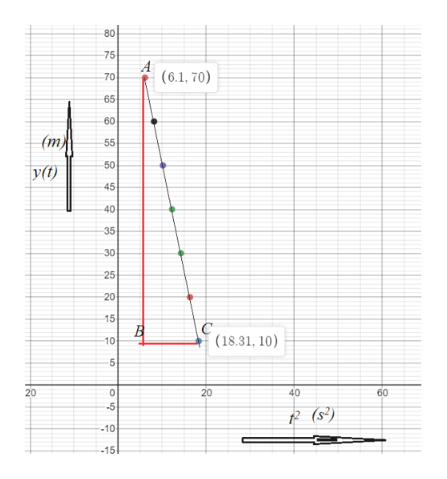# Data numberytt2110m4.28s18.31s220m4.42s16.32s330m3.78s14.28s440m3.50s12.25550m3.19s10.17s660m2.86s8.17s770m2.47s6.10sThe equation of distance as a function of time for a freely falling object is described, according to theory, by the equation:since (vi=0 , yi=0)y(t)= yi+vt*t+1/2*g*t2 Use the table for :make a plot of y(t) vs t2  , and then calculate the slope in order to find g (the acceleration due to gravity, which is  9.8 m/s2) find Slope =  Δy / Δt2g =

Question
37 views
 Data number y t t2 1 10m 4.28s 18.31s 2 20m 4.42s 16.32s 3 30m 3.78s 14.28s 4 40m 3.50s 12.25 5 50m 3.19s 10.17s 6 60m 2.86s 8.17s 7 70m 2.47s 6.10s

The equation of distance as a function of time for a freely falling object is described, according to theory, by the equation:

since (vi=0 , yi=0)

y(t)= yi+vt*t+1/2*g*t2

Use the table for :

• make a plot of y(t) vs t2  , and then calculate the slope in order to find g (the acceleration due to gravity, which is  9.8 m/s2)
•  find Slope =  Δy / Δt2
• g =
check_circle

Step 1

Plot the distance y(t) along y-axis and t2 along ...help_outlineImage Transcriptionclose80 75 A (6.1, 70 70 65 60 (m) 55 50 y(t) 45 40 35 30 25 15 B C (18.31, 10) 10 5 0 40 60 -5 -10 --15 20 20 20 fullscreen

### Want to see the full answer?

See Solution

#### Want to see this answer and more?

Solutions are written by subject experts who are available 24/7. Questions are typically answered within 1 hour.*

See Solution
*Response times may vary by subject and question.
Tagged in

### Physics